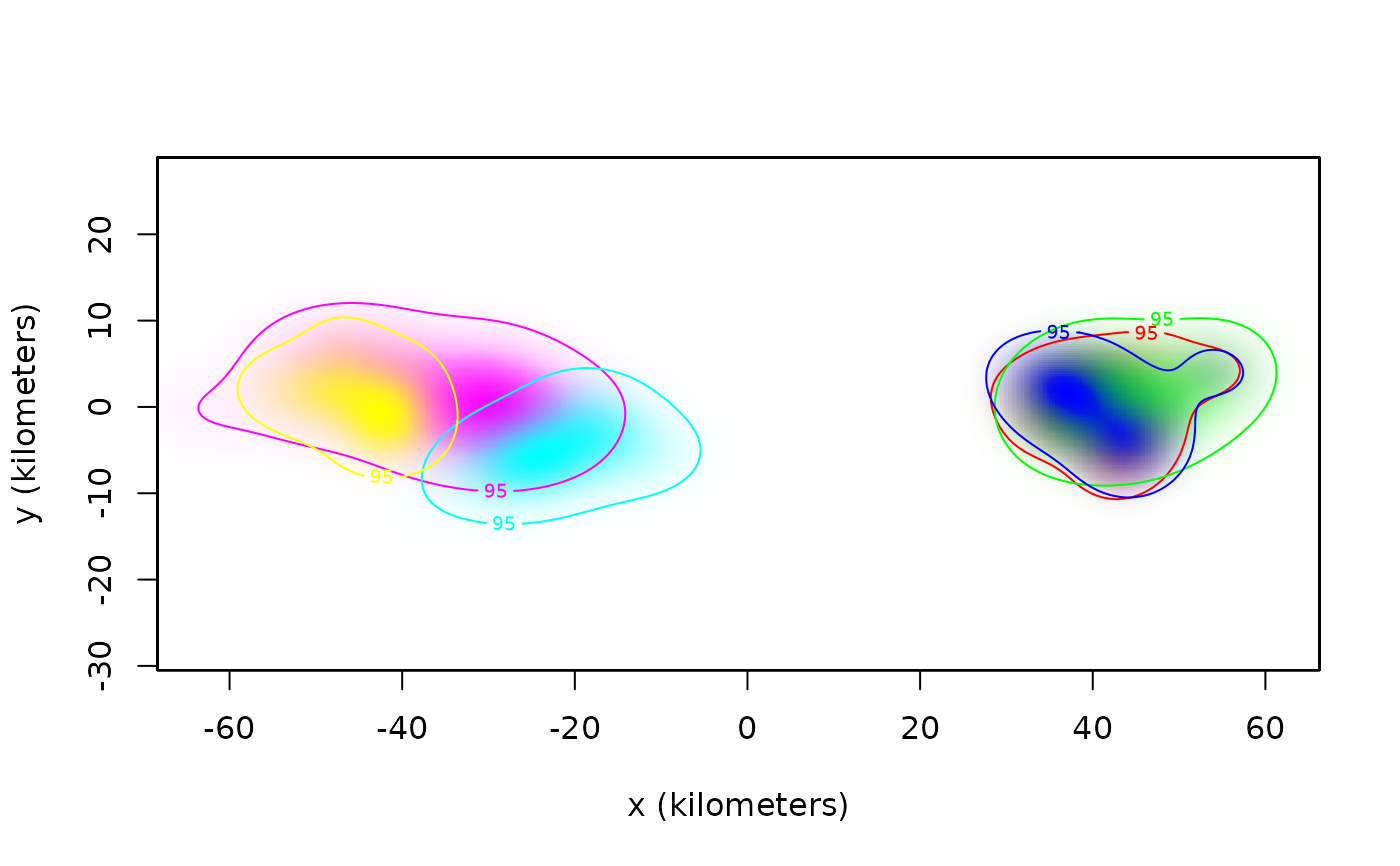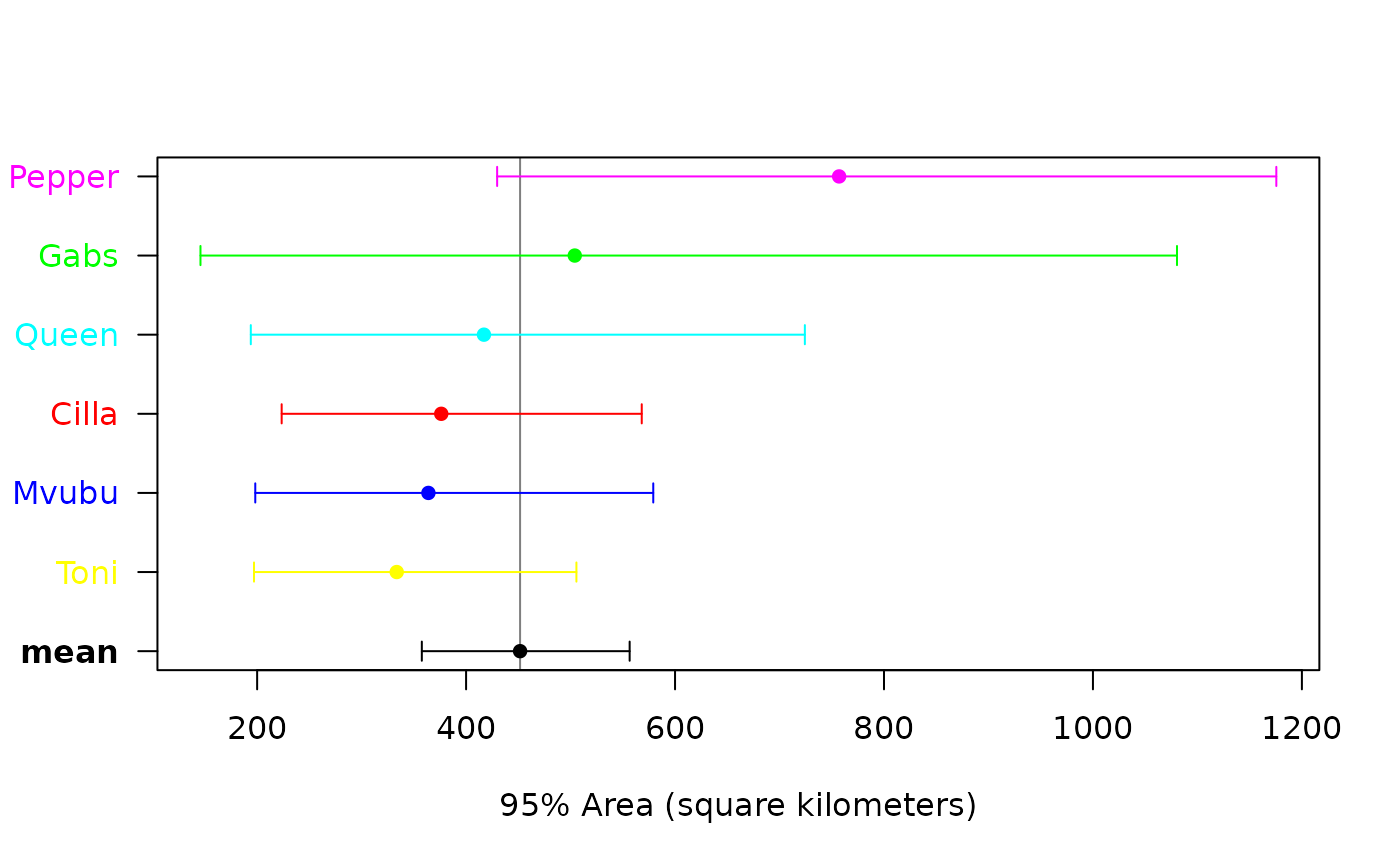These functions estimate population-level parameters from individual movement models and related estimates, including AKDE home-range areas, while taking into account estimation uncertainty.

meta(x,level=0.95,level.UD=0.95,method="MLE",IC="AICc",boot=FALSE,error=0.01,debias=TRUE,
verbose=FALSE,units=TRUE,plot=TRUE,sort=FALSE,mean=TRUE,col="black",...)

## Arguments

x A list of ctmm movement-model objects, UD objects, or UD summary output, constituting a sampled population, or a list of such lists, each constituting a sampled sub-population. Confidence level for parameter estimates. Coverage level for home-range estimates. E.g., 50% core home range. Statistical estimator used---either maximum likelihood estimation based ("MLE") or approximate best linear unbiased estimator' ("BLUE"). Information criterion to determine whether or not population variation can be estimated. Can be "AICc", AIC, or "BIC". Perform a parametric bootstrap for confidence intervals and first-order bias correction if debias=TRUE. Relative error tolerance for parametric bootstrap. Apply Bessel's inverse-Gaussian correction and various other bias corrections if method="MLE", REML if method="BLUE", and an additional first-order correction if boot=TRUE. Return a list of both cross-population and sub-population analyses if TRUE and x is a list of sub-population lists. Convert result to natural units. Generate a meta-analysis forest plot. Sort individuals by their point estimates in forest plot. Include population mean estimate in forest plot. Color(s) for individual labels and error bars. Further arguments passed to plot.

## Details

So-far only the meta-analysis of home-range areas is implemented. More details will be provided in an upcomming manuscript.

For both estimator methods, the same underlying model is assumed.

## Value

If x constitutes a sampled population, then meta returns a table with rows corresponding to the population mean and coefficient of variation. If x constitutes a list of sampled sub-populations, then meta returns confidence intervals on the sub-population mean ratios.

C. H. Fleming.

## Note

The AICc formula is approximated via the Gaussian relation.

akde, cluster, ctmm.fit.

## Examples

# \donttest{
library(ctmm)
data(buffalo)

# fit movement models
FITS <- AKDES <- list()
for(i in 1:length(buffalo))
{
GUESS <- ctmm.guess(buffalo[[i]],interactive=FALSE)
# use ctmm.select unless you are certain that the selected model is OUF
FITS[[i]] <- ctmm.fit(buffalo[[i]],GUESS,trace=2)
}
#> Maximizing likelihood.#> Calculating Hessian.#> Calculating REML gradient.#> Calculating REML Hessian.#> Maximizing likelihood.#> Calculating Hessian.#> Calculating REML gradient.#> Calculating REML Hessian.#> Maximizing likelihood.#> Calculating Hessian.#> Calculating REML gradient.#> Calculating REML Hessian.#> Maximizing likelihood.#> Calculating Hessian.#> Calculating REML gradient.#> Calculating REML Hessian.#> Maximizing likelihood.#> Calculating Hessian.#> Calculating REML gradient.#> Calculating REML Hessian.#> Maximizing likelihood.#> Calculating Hessian.#> Calculating REML gradient.#> Calculating REML Hessian.
# calculate AKDES on a consistent grid
AKDES <- akde(buffalo,FITS,trace=1)
#> Default grid size of 3 minutes chosen for bandwidth(...,fast=TRUE).#> Bandwidth optimization complete.#> Default grid size of 2 minutes chosen for bandwidth(...,fast=TRUE).#> Bandwidth optimization complete.#> Default grid size of 2 minutes chosen for bandwidth(...,fast=TRUE).#> Bandwidth optimization complete.#> Default grid size of 3 minutes chosen for bandwidth(...,fast=TRUE).#> Bandwidth optimization complete.#> Default grid size of 2 minutes chosen for bandwidth(...,fast=TRUE).#> Bandwidth optimization complete.#> Default grid size of 5 minutes chosen for bandwidth(...,fast=TRUE).#> Bandwidth optimization complete.
# color to be spatially distinct
COL <- color(AKDES,by='individual')

# plot AKDEs
plot(AKDES,col.DF=COL,col.level=COL,col.grid=NA,level=NA)# meta-analysis of buffalo
meta(AKDES,col=c(COL,'black'),sort=TRUE)
#>                     ΔAICc
#> Dirac-δ          0.000000
#> inverse-Gaussian 3.832925#>                  low      est     high
#> mean (km²)  357.5927 451.6346 556.4889
#> CoV² (RVAR)   0.0000   0.0000      Inf
#> CoV  (RSTD)   0.0000   0.0000      Inf# }`